# Problem 2: The uniform rod of length, L, and mass, m, shown below, is released from rest and from an angle of 8- O (radians]. The rod then begins to rotate clockwise due to the force of gravity m...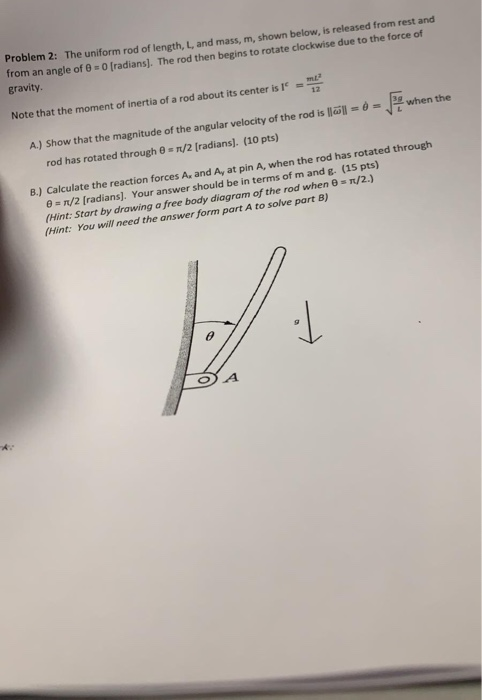Problem 2: The uniform rod of length, L, and mass, m, shown below, is released from rest and from an angle of 8- O (radians]. The rod then begins to rotate clockwise due to the force of gravity mE 12 Note that the moment of inertia of a rod about its center is when the A) Show that the magnitude of the angular velocity of the rod is löll- rod has rotated through θ s n/2 [radians]. (10 pts) forces A, and A, at pin A, when the rod has rotated through B.) Calculate the reaction e π/2 [radians (Hint: Start by drawing a free body diagram of (Hint: You will need the answer J. Your answer should be in terms of m and g. (15 pts) of the rod when rt A to solve part B) T/2.) A.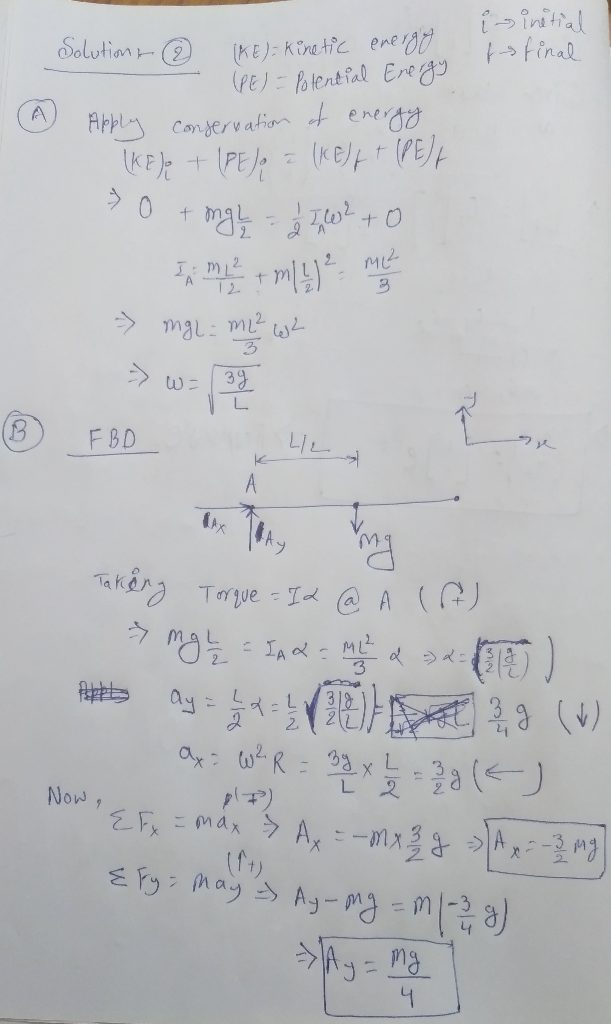##### Add Answer of: Problem 2: The uniform rod of length, L, and mass, m, shown below, is released from rest and from an angle of 8- O (radians]. The rod then begins to rotate clockwise due to the force of gravity m...
Similar Homework Help Questions
• ### A uniform rod of mass M and length L is released from its horizontal position. The...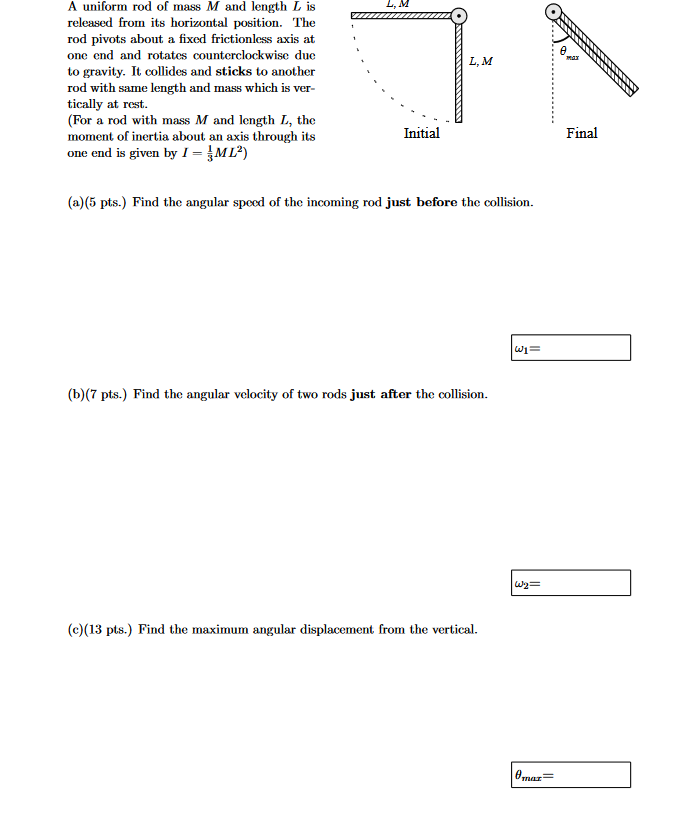A uniform rod of mass M and length L is released from its horizontal position. The rod pivots about a fixed frictionless axis at' onc end and rotates countcrclockwise duc to gravity. It collides and sticks to another rod with same length and mass which is ver- tically at rest. (For a rod with mass M and length L, the moment of inertia about an axis through its one end is given by1-ML) L,M L, M Initial Final (a)(5 pts.)...

• ### )y 4. The figures to the right show a rod with length, l, and mass, M, on a frictionless table rotated an angle θ from the horizontal. It is fix to the table by a pin Sring through its center of mass...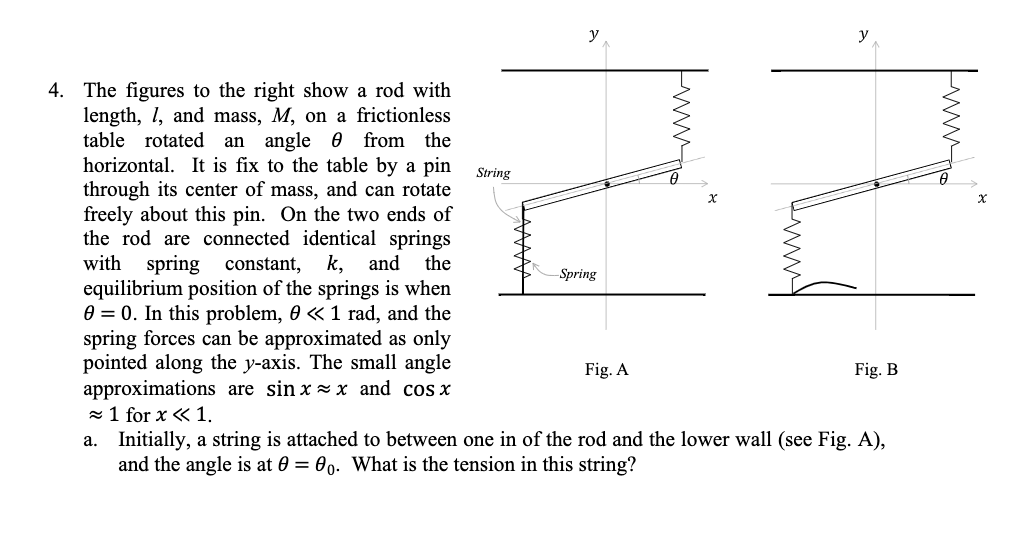)y 4. The figures to the right show a rod with length, l, and mass, M, on a frictionless table rotated an angle θ from the horizontal. It is fix to the table by a pin Sring through its center of mass, and can rotate freely about this pin. On the two ends of the rod are connected identical springs ith spring constant, k, and the equilibrium position of the springs is when θ-0. In this problem, θ 1 rad,...

• ### A slender 9 lb rod can rotate in a vertical plane about a pivot at B. A spring of constant k-30 lb/ft and of unstretched length 6 in. is attached to the rod as shown. The rod is released from rest in...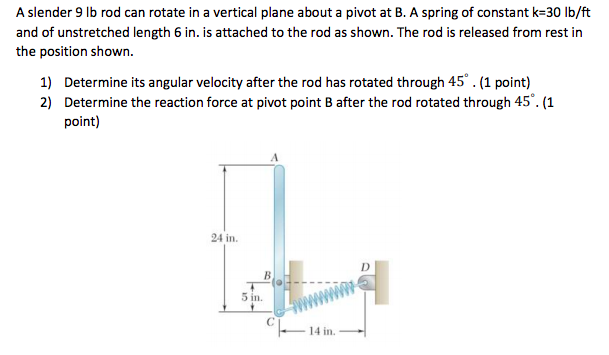A slender 9 lb rod can rotate in a vertical plane about a pivot at B. A spring of constant k-30 lb/ft and of unstretched length 6 in. is attached to the rod as shown. The rod is released from rest in the position shown. 1) Determine its angular velocity after the rod has rotated through 45.(1 point) 2) Determine the reaction force at pivot point B after the rod rotated through 45. (1 point) 24 in 5 in. 4...

• ### The uniform bar shown in the diagram has a length of 0.80 m. The bar begins to rotate from rest in the horizontal...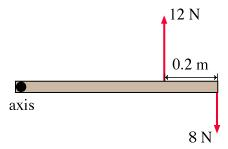The uniform bar shown in the diagram has a length of 0.80 m. The bar begins to rotate from rest in the horizontal plane about the axis passing through its left end. What will be the magnitude of the angular momentum L of the bar 6.0 s after the motion has begun? The forces acting on the bar are shown. Express your answer in kg*m2/s

• ### Sample Problem 6. The 30 kg slender rod is released from rest when θ-00 (when rod OA is in the horizontal position). The spring is unstretched at θ-00. A constant clockwise moment of 10 N-m is applie...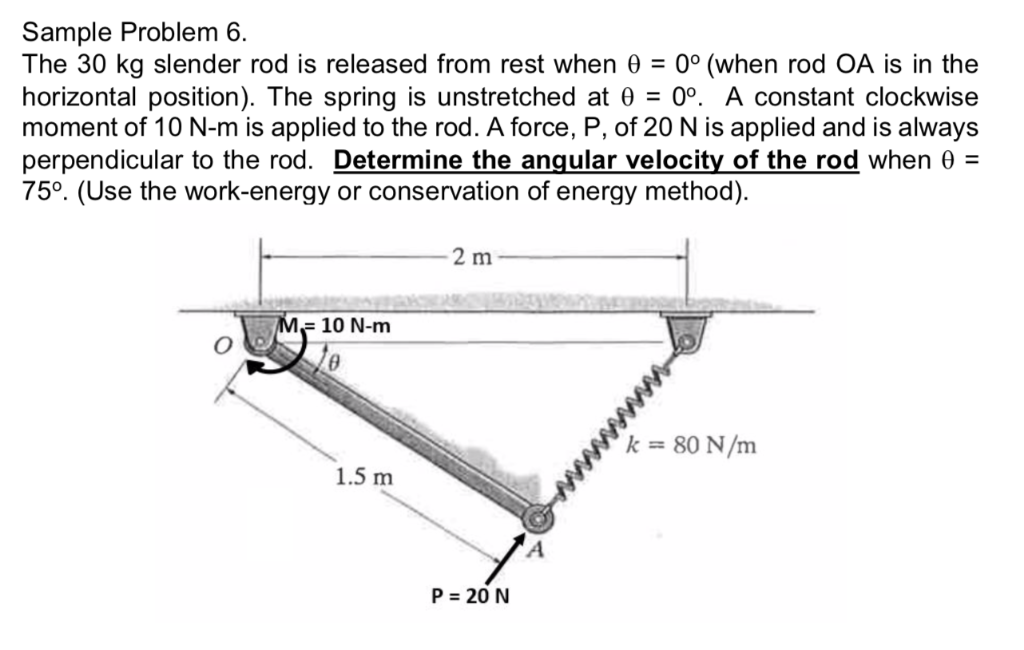Sample Problem 6. The 30 kg slender rod is released from rest when θ-00 (when rod OA is in the horizontal position). The spring is unstretched at θ-00. A constant clockwise moment of 10 N-m is applied to the rod. A force, P, of 20 N is applied and is always perpendicular to the rod. Determine the angular velocity of the rod when θ- 750. (Use the work-energy or conservation of energy method). 2 m 10 N-mm k80 N/m 1.5...

• ### A uniform rod with a mass of m = 1.73 kg and a length of l...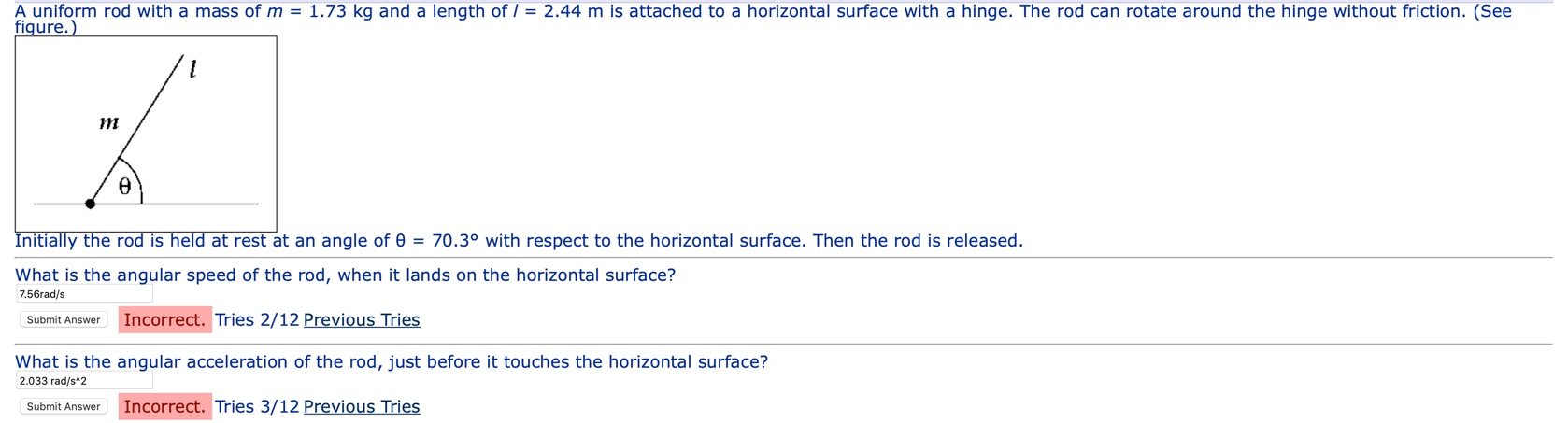A uniform rod with a mass of m = 1.73 kg and a length of l = 2.44 m is attached to a horizontal surface with a hinge. The rod can rotate around the hinge without friction. (See figure.) Initially the rod is held at rest at an angle of 0 = 70.3° with respect to the horizontal surface. Then the rod is released. What is the angular speed of the rod, when it lands on the horizontal surface? 7.56rad/s...

• ### Q19: The uniform rod of mass M- 2 kg and length of L- 8 cm is...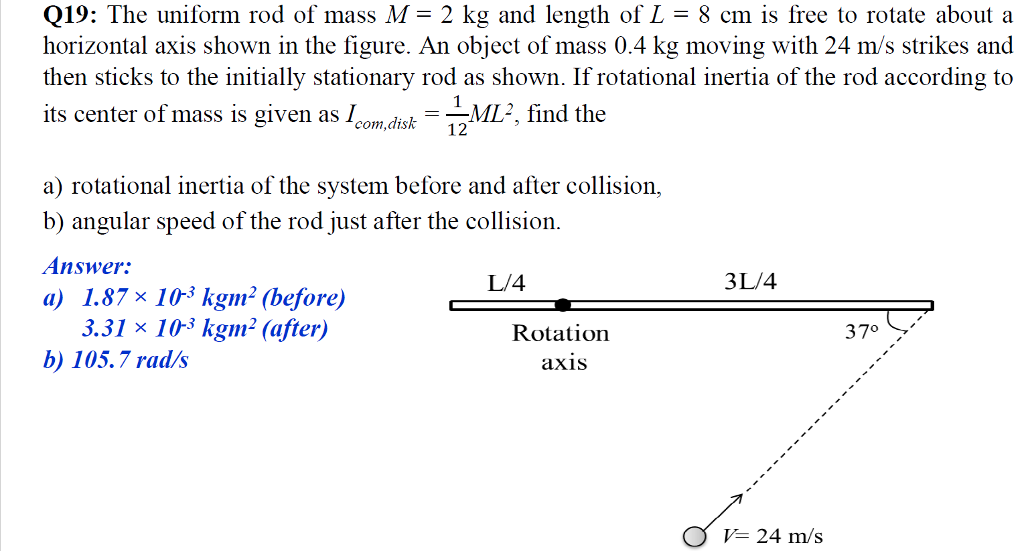Q19: The uniform rod of mass M- 2 kg and length of L- 8 cm is free to rotate about a horizontal axis shown in the figure. An object of mass 0.4 kg moving with 24 m/s strikes and then sticks to the initially stationary rod as shown. If rotational inertia of the rod according to its center of mass is given as Icmdisk M, find the a) rotational inertia of the system before and after collision, b) angular speed...

• ### A uniform thin rod of length 0.65 m and mass 3.4 kg can rotate in a horizontal plane about a vert...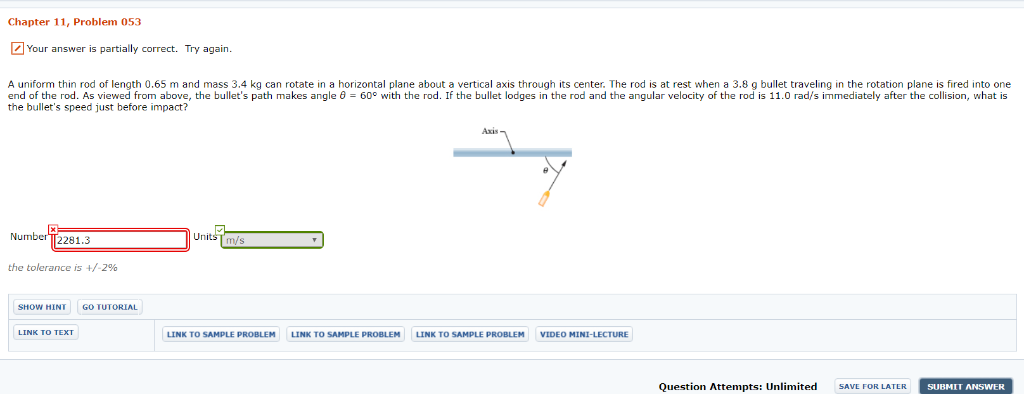A uniform thin rod of length 0.65 m and mass 3.4 kg can rotate in a horizontal plane about a vertical axis through its center. The rod is at rest when a 3.8 g bullet traveling in the rotation plane is fired into one end of the rod. As viewed from above, the bullet's path makes angle θ = 60° with the rod. If the bullet lodges in the rod and the angular velocity of the rod is 11.0 rad/s...

• ### Q-4 (25 pts A uniform bar of length (L), cross sectional area (A) and mass (m) is free to rotate about a frictionle...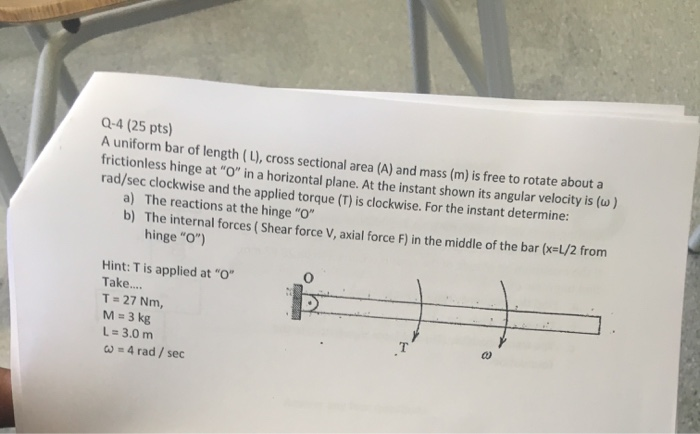Q-4 (25 pts A uniform bar of length (L), cross sectional area (A) and mass (m) is free to rotate about a frictionless hinge at "O" in a horizontal plane. At the instant shown its angular velocity is (w) rad/sec clockwise and the applied torque (T) is clockwise. For the instant determine: a) The reactions at the hinge "O b) The internal forces (Shear force V, axial force F) in the middle of the bar (xeL/2 from hinge "O") Hint:...

• ### Problem3 A uniform slender rod of length L- 2 m and mass 3 ke rotates freely nodty soUARED S hinge at A. At t-0, the initial angle is θ-30 and the in rads/s in the counter-clockwise direction....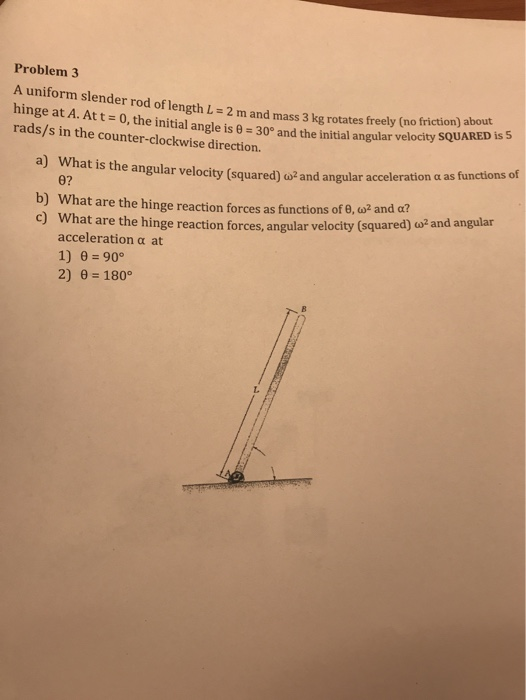Problem3 A uniform slender rod of length L- 2 m and mass 3 ke rotates freely nodty soUARED S hinge at A. At t-0, the initial angle is θ-30 and the in rads/s in the counter-clockwise direction. (no friction) about itial angular velocity SQUARED is 5 What is the angular velocity (squared)d02 and angular acceleration α as functions of 0? a) b) What are the hinge reaction forces as functions of 0, (u? and a? c) What are the hinge...

Need Online Homework Help?